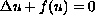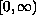Electron. J. Diff. Eqns., Vol. 1999(1999), No. 43, pp. 1-16.

### Bifurcations for semilinear elliptic equations with convex nonlinearity J. Karatson & P. L. Simon

Abstract:
We investigate the exact number of positive solutions of the semilinear Dirichlet boundary value problemon a ball in Rn where f is a strictly convex C2 function on. For the one-dimensional case we classify all strictly convex C2 functions according to the shape of the bifurcation diagram. The exact number of positive solutions may be 2, 1, or 0, depending on the radius. This full classification is due to our main lemma, which implies that the time-map cannot have a minimum. For the case n>1 we prove that for sublinear functions there exists a unique solution for all R. For other convex functions estimates are given for the number of positive solutions depending on R. The proof of our results relies on the characterization of the shape of the time-map.

Submitted June 22, 1999. Published October 18, 1999.
Math Subject Classifications: 35J60.
Key Words: semilinear elliptic equations, time-map, bifurcation diagram.

Show me the PDF file (174K), TEX file, and other files for this article.Janos Karatson Department of Applied Analysis Eotvos Lorand University Budapest, Hungary e-mail: karatson@cs.elte.huPeter L. Simon Department of Applied Analysis Eotvos Lorand University Budapest, Hungary e-mail: simonp@cs.elte.hu